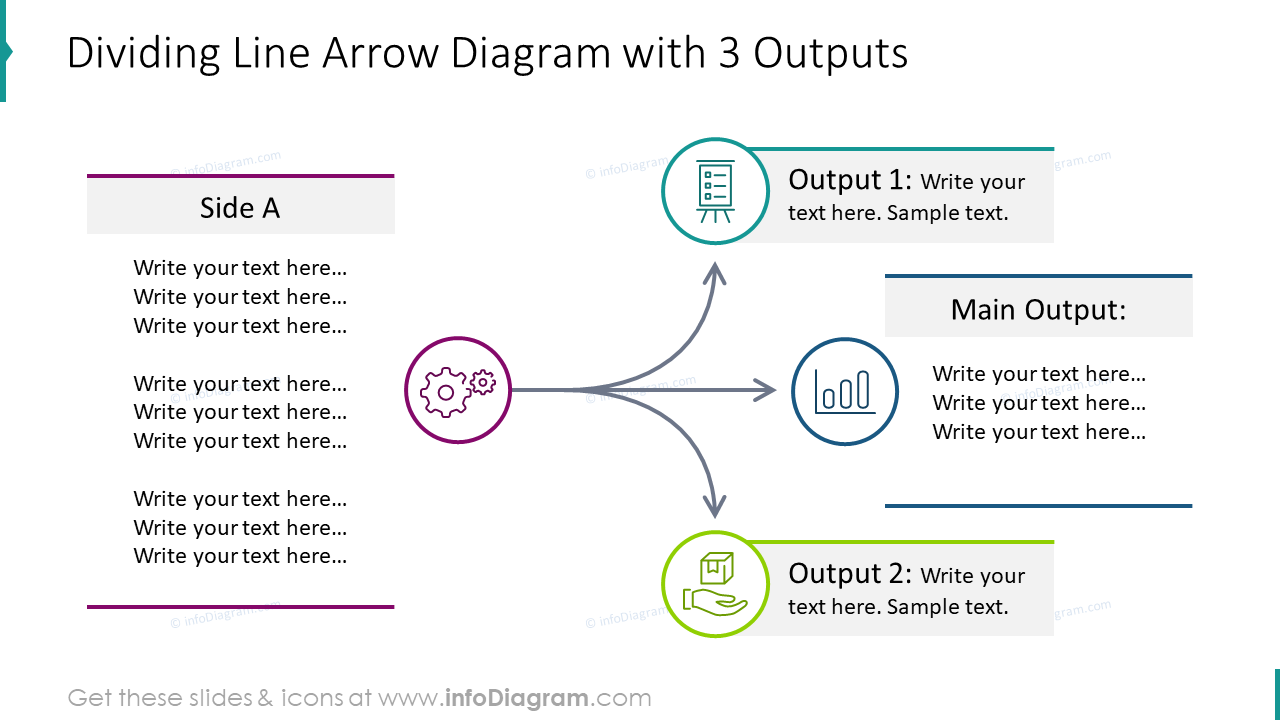# Dividing line arrow diagram with three outputs from deck Outline Diagrams - Ultimate Deck for Visual Presentations (PPT graphics)Slide contents:

• diagramHow
• diagramWhere
• 3_items
• 4_items
• Outline
• outline diagrams
• diagram
• diagrams set
• arrow
• divide
• dividing arrow diagram
• outputs

### Related products

PowerPoint Templates